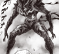## Resources for Farrow/Transpose farrow filter design.Started by 2 months ago4 replieslatest reply 1 month ago139 views

Hi, I want to design transpose farrow filter for sample rate conversion. There are some blogs explaining how it works on internet and on dsprelated.com as well, but I found only one with detailed design process and matlab code (link). I don't have matlab optimization toolbox, therefore I implemented matlab code from the document in python, scipy.optimize.linprog is used to solve linear program problem. To check my implementation I used example 1 from Matlab code section of the document but the outputs are not matching. I debugged my python implementation and made sure that the input matrix, vectors to a linprog

matches to the matlab version. can you please point me to any more resources/examples/tutorial/code for

farrow/transpose farrow filter design, filter design using linear programming etc.

Thanks,

Umesh.

[ - ]Umesh,

A good reference on piecewise polynomial interpolators, including Farrow, is Digitial Communications, a Discrete-Time Approach, by Michael Rice, section 8.4.2.

regards,

Neil

[ - ]Thank you, I will take a look at this.

[ - ]If you mean fractional delay rather than sample rate change. This matlab model may be easier to understand as it applies each polyphase separately then adds up as per second order transpose structure. The function "filter" is based on convolution:

h = [1/2,-1/2,0; -1,0,1; 1/2,1/2,0]; %coefficients, 2nd order Farrow

d = 0.7; %delay

x = randn(1,1000); %random input

y = (filter(h(:,1),1,x) * d + filter(h(:,2),1,x)) * d + filter(h(:,3),1,x);

plot(x,'.-'); hold;

plot(y,'r.-');

[ - ]